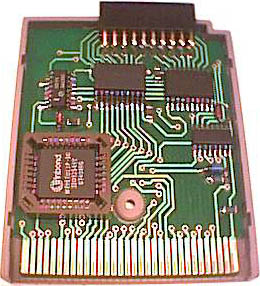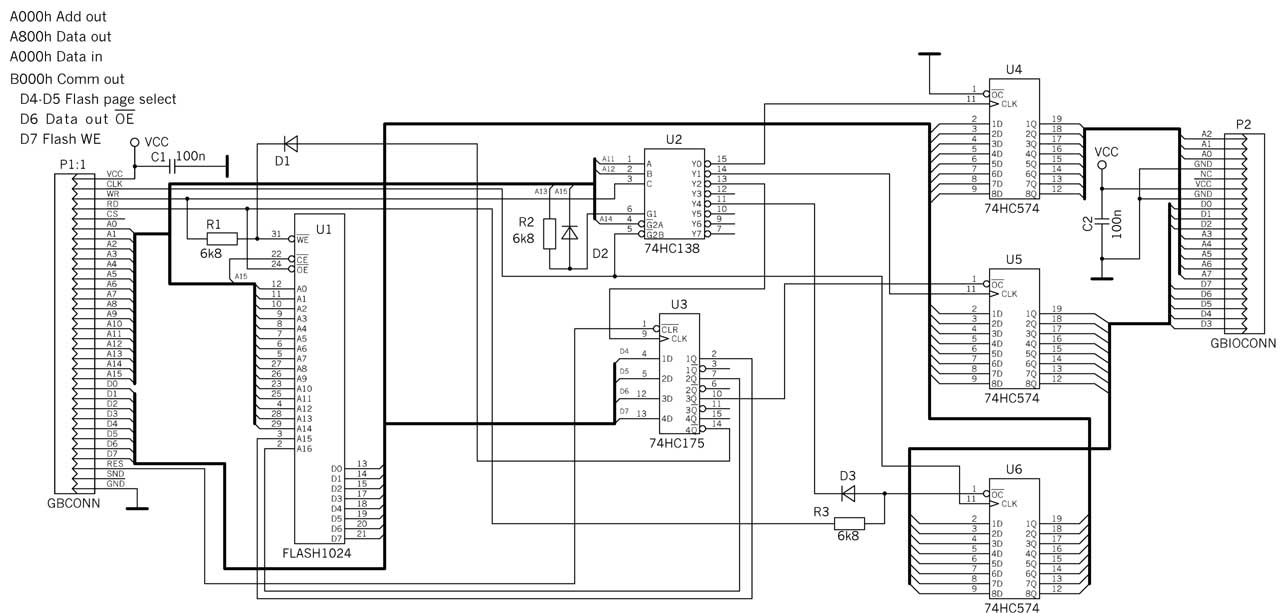<GoCom>  <Gondos Csaba> <HG5BMU>

Gameboy Basic I/O cartridgeGB-Basic ROM program V2.5: Write mail

GB Basic V2.10 Documentation

GB Basic is a floating-point BASIC interpreter for GameBoy that allows you to write & execute programs up to 7.4K bytes in size. If it is used in a cart with RAM then a program can be SAVEd and LOADed at a later time.  Currently string variables are not supported, but may be in the future. Long variable names are not supported. Variable names may bea letter or a letter and a number.  Each line requires a line number.  &H may be used for entering hex values. (Ex: POKE &HFF00,&HFF)

The following commands are supported:

ABS(x)         -       Return the absolute value of x.

AUTO          -       Enable Auto load & run program on reset.

CLEAR        -       Clear all variables.

CLS              -       Clear the screen

COLOR x      -       Set drawing color to x. 0=Black, 1=Dark Gray, 2=Light Gray, 3=White, & 4=XOR.

(Defaults to XOR on program execution.)

COS(x)          -       Return the cosine of x given in radians.

DIM              -       Only single dimension arrays are currently supported.

If not explicitly defined by a DIM statement, it is assumed to be a size of 10 upon first reference.

DATA x,...      -       Data in program.

DELAY x        -       Delay from 10ms to 655 seconds.  (Delay length = x * 10ms)

END               -       End program.

FOR/NEXT     -       Program loop.

FREE               -       Display remaining amount of free memory.

GOSUB           -       Execute a subroutine.

GOTO             -       Goto specific line.

IF/THEN          -       Conditional execution.

INPUT             -       Input data from user.

INT(x)              -       Returns the integer of x.

KEYPAD(x)      -       If x=0, the following bits are returned if that button is pressed. 128 - Start    8 - Down  64 - Select

4 - Up  32 - B   2 - Left   16 - A   - Right    If x>0, then x is ANDed with the bits above.

If the result = zero, 0 is returned.   If result <> zero, a 1 is returned.

LET                    -       Assign a value to a variable. (Optional)

LINE x1,y1,x2,y2 -       Draw a line on the screen using COLOR.   (0<=x<=127,0<=y<=119)

Link 2 - Configure port for external keyboard.

LIST [x][-][x]       -       List lines of the program. Starting and/or ending line numbers are optional.

LOAD n               -       Load program from battery-backed RAM.(n- program number in flash memory V2.5)

LOCATE y,x       -       Position cursor with coordinates y,x.

MEMTOP x         -       Set Last address of RAM for GB Basic to use.  On power up or reset this defaults to \$dfff.

Lowering this value reserves memory for USR for example. NOTE: All variables are

CLEARed when this instruction is executed.

NEW                  -       Erase all program lines in memory.

PEEK(x)              -       Return value of a memory location x.

POINT x,y            -       Draw a point on the screen using COLOR.  (0<=x<=127,0<=y<=119)

POKE x,y             -       Write value y to a memory location x.

PRINT                -       Print to screen.

REG x,y              -       Sets value of cpu BC register (x) and cpu    DE register (y) for entry into USR routine.

REM                  -       Remark. Also ' is supported.

RENUM            -       Renumber program.

RESTORE          -       Set DATA pointer to a specific line number. If  no line number present, beginning of program.

RETURN           -       Return from subroutine.

RND(x)               -       Returns a random number between 0 and 1. x is ignored.

RUN                  -       Execute program.

SAVE n               -       Save program to battery-backed RAM.(n- program number in flash memory V2.5)

SCREEN x         -       If x=0, set text mode. If x=1, set graphics mode.

SERVO x,y        -       Controls the position of up to 8 memory-mapped servos. SERVO 0,a defines the memory address of

the servos output port and enables the servo interrupt routine. SERVO x,y outputs a high

pulse to servo x for 693+(y*7.6) microseconds  giving a pulse range of .7 to 2.3 ms. A value of

y = 0 disables the output pulse of that servo. This pulse is sent out every 20 ms. Servos may be

set before issuing SERVO 0,a to enable them. On  reset, servos default to off (y=0) and disabled.

NOTE: When enabled, servos slow down system about  15% due to servo interrupt servicing.

Use SERVO 0,0 to disable all servos once they have been enabled. (1<=x<=8,0<=y<=210)

SGN(x)               -       Returns 1 if x > or = 0. Else returns value -1.

SIN(x)               -       Return the sine of x given in radians.

SINIT x,y            -       Serial Initialize: This command has 3 functions:    1) Set MBC serial output baud rate. (x)

2) Set MBC digital output pins. (y)    3) Set the polarity of MBC serial out. (bit 0 of y)

(SERIAL IS OUTPUT ON AN MBC PORT PIN. NOT ON THE  GB LINK PORT CONNECTOR.)

Baud Rate values for x:  0 = 1200 (Only available in SPEED 0 mode.) 1 = 2400 2 = 4800  3 = 9600

4 = 14400 5 = 19200  6 = 38400   7 = 57600 (Only available on GBC in SPEED 1 mode.)

Digital Outputs bits:   MBC1: 0=Pin6, 1=Pin7  MBC5: 0=Pin29, 1=Pin28, 2=Pin27, 3=Pin26

For serial output:   If bit 0 of y = 0, serial idle level is low.     If bit 0 of y = 1, serial idle level is high.

SOUT x           -       Serial output value x on MBC output port pin.    For MBC1, serial output on pin 6 of MBC1.

For MBC5, serial output on pin 29 of MBC5.

(SERIAL IS OUTPUT ON AN MBC PORT PIN. NOT ON THE  GB LINK PORT CONNECTOR.)

SOUND x,y        -       Generate a sound of frequency x for y * 10 milli- seconds. If y=65535, sound will stay on infinitely.

If y=0, sound is turned off.

SPEED x          -       x=0, Set single speed mode. (Default on powerup.)  x=1, Set double speed mode.

(Only has an effect on GB Colo(u)r.

SQR(x)               -       Returns the square root of x.

STEP                 -       Used with FOR for specifying increment.

STOP                 -       Same as end but displays line number last executed.

TAB(x)               -       Used with PRINT command for moving cursor to column x.

TAN(x)               -       Returns the tangent of x given in radians.

USR(x)               -       Call user assembly language program at address x. Return value reflects cpu HL registers contents.

Revision History:

V2.5    by GoCom     Fixed bug INT(x) -1>x>1  Save and Load to Flash Eprom   Print character to graphics screen